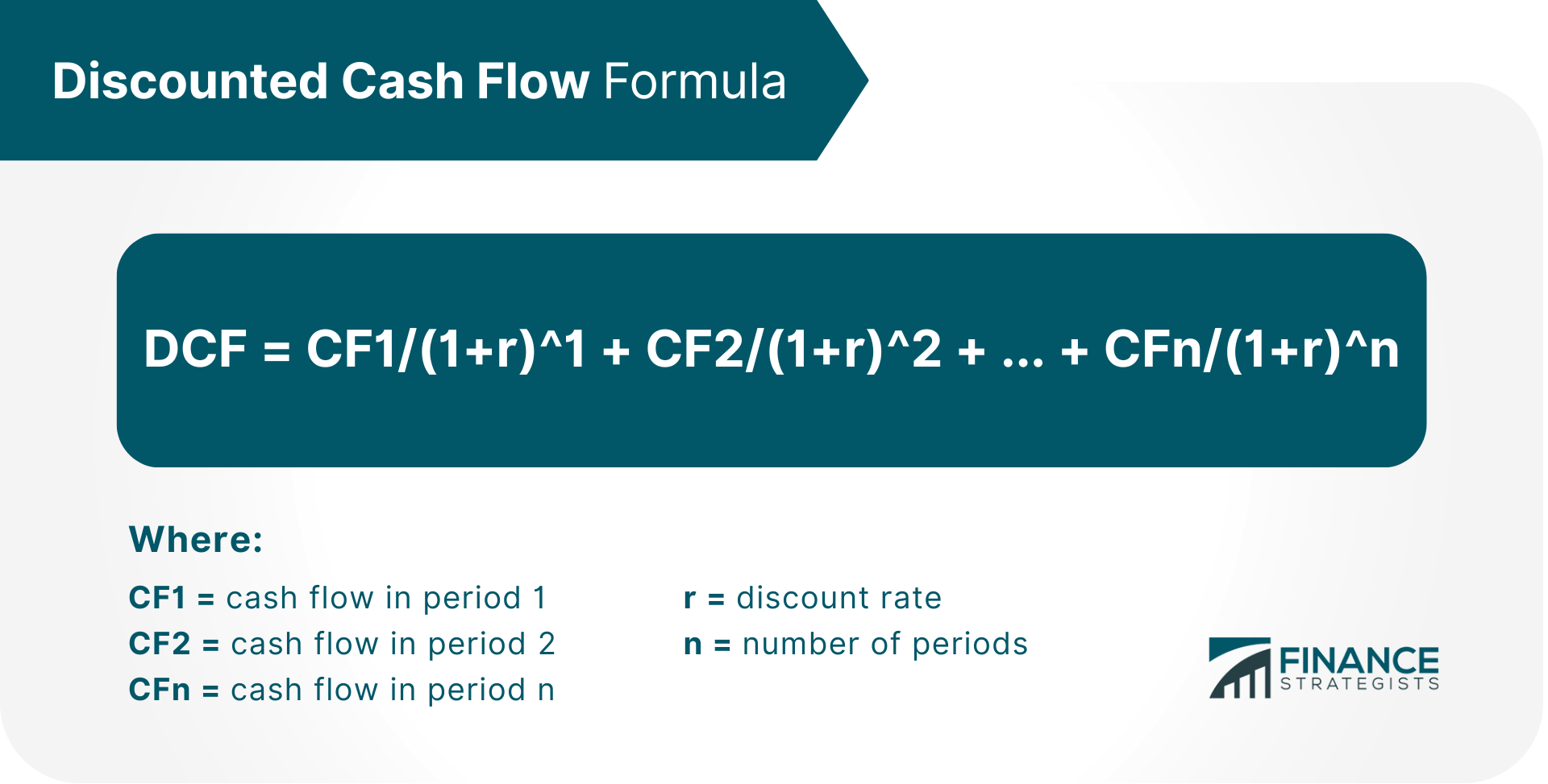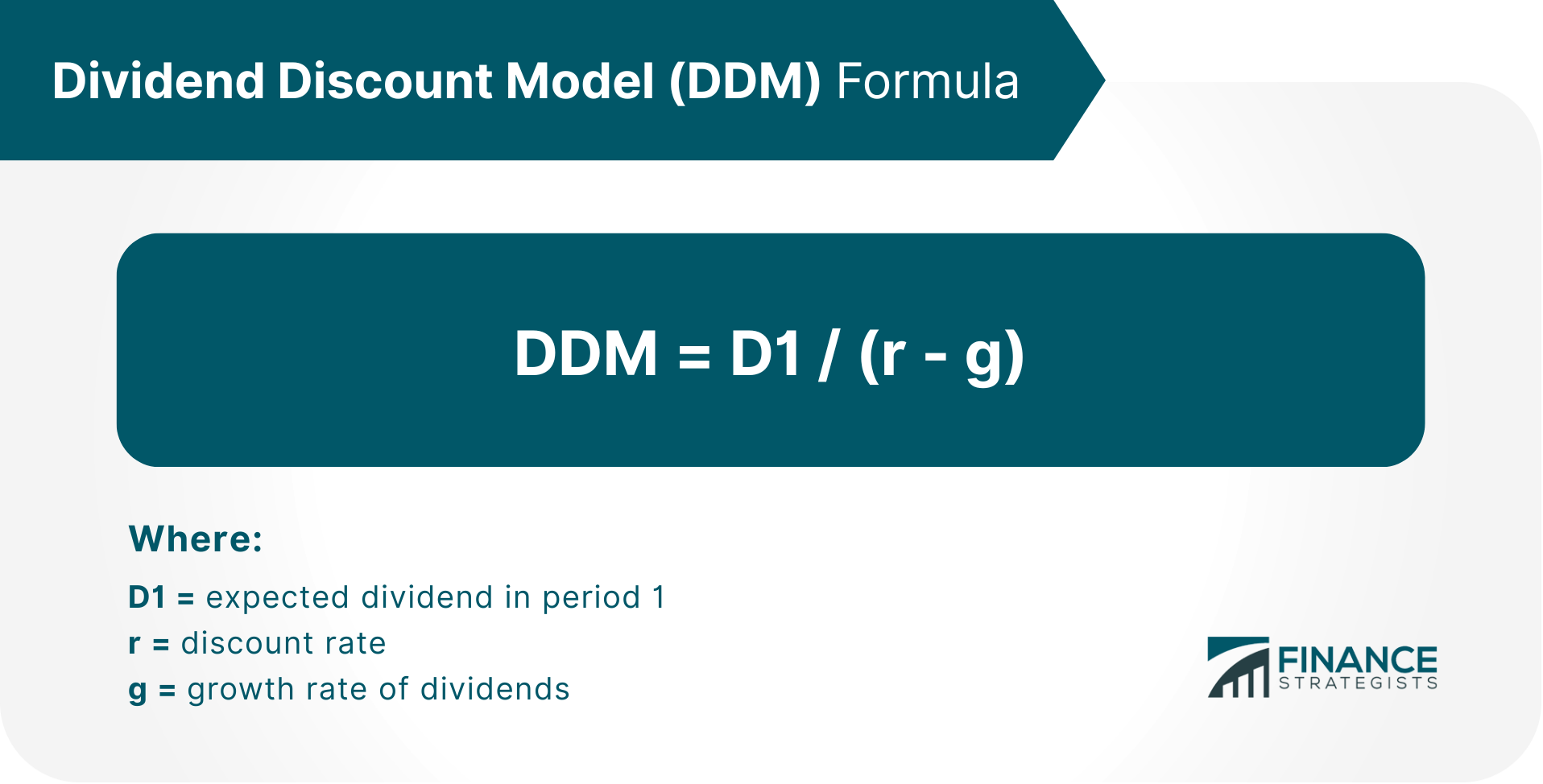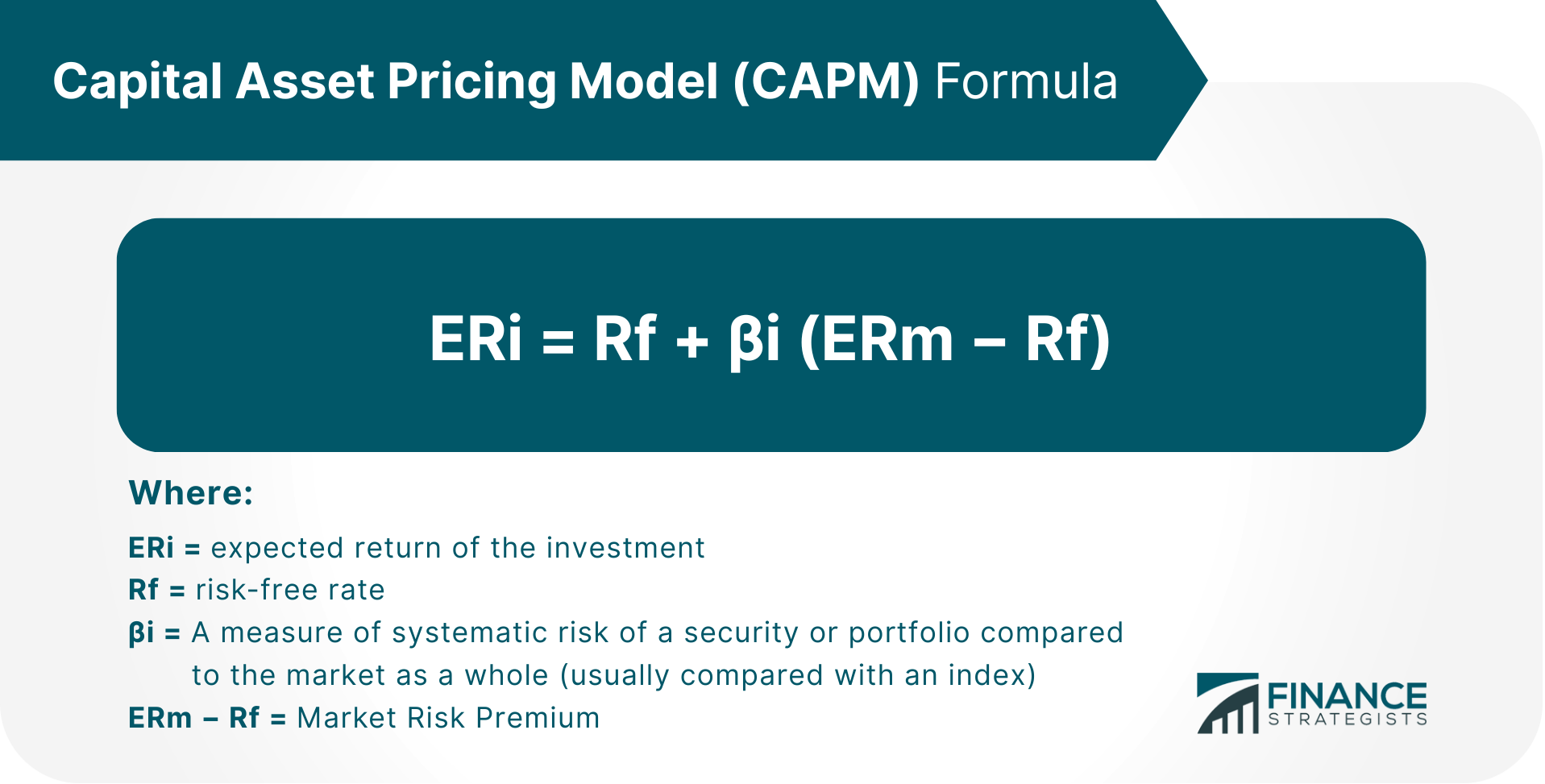# Discounted Cash Flow Model### Written byTrue Tamplin, BSc, CEPF® | Reviewed by Editorial Team

Updated on December 28, 2022

## What Is the Discounted Cash Flow (DCF) Model?

The Discounted Cash Flow (DCF) model is a valuation method used to estimate the intrinsic value of a company. The model is based on the principle that the value of a business is equal to the present value of its future cash flows.

In other words, the DCF model discounts a company's expected cash flows in order to arrive at a present value that reflects the time value of money. This present value can then be compared to the current market price of the stock in order to determine whether it is under- or overvalued.

Discounting cash flows is important because it takes into account the fact that money today is worth more than money in the future. This is due to the time value of money, which states that a dollar today is worth more than a dollar in the future because the dollar can be invested and will grow over time.

The DCF model is a powerful tool for valuing companies, but it also has its limitations. In particular, the model relies on a number of assumptions that may not always hold true in the real world.

## Discounted Cash Flow Formula

The discounted cash flow (DCF) formula is as follows:## Understanding the Components of the DCF Formula

There are three main components to the DCF formula: cash flows, the discount rate, and the number of periods.

### Cash Flow (CF)

The cash flow that is being discounted can be from any source, such as earnings, dividends, or even cash that will be generated from the sale of an asset.

For example, if a company is looking to invest in a new factory, the cash flow from that investment would be the revenue generated from selling the products produced by the factory.

### Discount Rate (r)

The discount rate is the rate at which future cash flows are discounted back to their present value. The discount rate is often set at the investor's required rate of return, which is the minimum return that they require in order to invest in a company.

The discount rate can also be set at the weighted average cost of capital (WACC), which is the average of a company's cost of debt and cost of equity.

### Number of Periods (n)

The number of periods is the number of years over which the cash flows are expected to occur. It is often set at 10, which is the average life expectancy of a company.

## Expanding on How the DCF Model is Used

There are many ways in which the DCF model can be used. The model can be used to value a company as a whole, or it can be used to value specific assets such as patents or real estate. Common uses include the following:

### Equity Research

DCF analysis is also commonly used in equity research. Equity analysts use the DCF model to estimate the fair value of a company’s stock.

In general, equity analysts begin by estimating the future cash flows that the company is expected to generate. These cash flows are then discounted back to present value, and the resulting figure is divided by the number of shares outstanding. This figure is known as the intrinsic value per share, and it represents the analyst’s estimate of the fair value of the stock.

For example, ABC's current stock price is \$144.87 per share. Assuming ABC will generate annual cash flows of \$30 billion for the next 5 years and we set a discount rate of 10%, we can calculate the intrinsic value per share as follows:

Year 1 = 30B/1+0.10^1 = 30B/1.1 = \$27.27B
Year 2 = 30B/1+0.10^2 = 30B/1.21 = \$24.79B
Year 3 = 30B/1+0.10^3 = 30B/1.331 = \$22.53B
Year 4 = 30B/1+0.10^4 = 30B/1.4641 = \$20.49B
Year 5 = 30B/1+0.10^5 = 30B/1.6105 = \$18.62B

We then add every year’s present value cash flows to get the total present value:

Total Present Value = \$27.27B + \$24.79B + \$22.53B + \$20.49B + \$18.62B = \$113.72B

We then divide the total present value by the number of shares outstanding (1,000,000,000 in this example) to get the intrinsic value per share:

Intrinsic Value per Share = \$113.72B/1,000,000,000 = \$113.72

As we can see, the intrinsic value per share is much lower than the current stock price. This suggests that ABC stock may be overvalued.

### Capital Budgeting

The DCF model can be used in capital budgeting, which is the process of making investment decisions. In particular, the DCF model can be used to evaluate whether a proposed investment is likely to generate sufficient returns.

Net Present Value (NPV) is the difference between the initial cost of an investment and its present value. In assessing the profitability of an investment using the DCF model, the resulting DCF should be higher than the initial cost for such investment to be considered profitable. If it is otherwise, it could signal that the investment is likely to be unprofitable and should be avoided.

For example, suppose a company is considering investing in a new factory. The company would need to estimate the future cash flows that the factory is expected to generate. These cash flows would then be discounted back to present value, and the resulting figure would be compared to the cost of the investment.

We can see this play out below:

The cost of an investment is \$1 million and the company estimates that the factory will generate annual cash flows of \$400,000 for the next 3 years. Discount rate is set at 10%.

The present value of the cash flows can be calculated as follows:

Year 1 = 400,000/1+0.10^1 = 400,000/1.1 = \$363,636
Year 2 = 400,000/1+0.10^2 = 400,000/1.21 = \$330,578
Year 3 = 400,000/1+0.10^3 = 400,000/1.331 = 300,525

Total Present Value/DCF = \$363,636 + \$330,578 + \$300,525 = \$994,739

Since the total present value or DCF is less than the cost of the investment, we can conclude that the investment is not worthwhile.

### Mergers and Acquisitions

The DCF model is also commonly used in M&As, more specifically in acquisitions

It involves analyzing the value of a potential acquisition based on both the price paid and other factors such as expected synergies between the two businesses involved.

In the case of a merger, the DCF model is used to determine the value of each company and how much they should be worth together. The model can also decide whether or not it is more beneficial for one company to acquire another or if it would make sense for both businesses to merge.

By estimating the intrinsic value of the company being acquired, and then comparing it to the price being paid, investors can get a better sense of whether or not an acquisition is a good deal.

## Pros of the Discounted Cash Flow Model

The DCF model is often seen as the most accurate way to value a company because it takes into account all of the cash that a company is expected to generate over its lifetime and applies a discount to account for the time value of money.

In contrast, other valuation methods such as the price-to-earnings (P/E) ratio only consider a company's historical earnings.

### Useful Barometer of a Company’s Objective Value

The DCF model is also often seen as the most useful indicator of a company's objective value. This is because the DCF model considers all of the factors that affect a company's value, such as its growth potential and riskiness.

### Adjustable to Each Investor’s Expectations

Another advantage of the DCF model is that it can be adjusted to each investor's expectations. For example, if an investor requires a higher return, they can simply adjust the discount rate accordingly.

### Provides Insight Into a Company’s Future Performance

The DCF model can provide valuable insight into a company's future performance. This is because the DCF model estimates a company's value based on its expected cash flows. As a result, the DCF model can be used to predict how a company's stock price will move in the future.

### Applicable to a Wide Variety of Investments, Projects, or Companies

The DCF model can be used to value a wide variety of investments, projects, or companies. This makes the DCF model a very versatile tool that can be used in many different situations.

### Suitable for Analyzing Mergers and Acquisitions

The DCF model is also well-suited for analyzing mergers and acquisitions. This is because the DCF model can be used to estimate the intrinsic value of both companies involved in the transaction.

## Cons of the Discounted Cash Flow Model

Much like any other valuation method, the DCF model was designed for a specific application with certain assumptions, which means it has a few limitations that you need to be aware of.

### Sensitivity to Assumptions

The DCF model is very sensitive to assumptions. This means that small changes in assumptions can have a big impact on the results of the DCF analysis.

For example, if we assume that XYZ Corp will grow at a rate of 5% per year instead of 10%, the intrinsic value of the company would be much higher:

DCF = \$100 million / (1 + 5%)^1

= \$95.24 million

DCF = \$100 million / (1 + 10%)^1

= \$90.91 million

### Does Not Consider Relative Valuations of Competitors

Another limitation of the DCF model is that it does not consider the relative valuations of competitors. This means that a company may be undervalued relative to its peers even if its intrinsic value is higher.

### Terminal Value Contribution

The DCF model also relies heavily on the terminal value, which is the value of a company at the end of the forecast period. The terminal value is usually estimated using a multiple of earnings or cash flow. However, these estimates can be flawed and may not reflect the true value of the company because they are based on assumptions.

### Difficulty to Assess the Correct Discount Rate to Use

Another challenge when using the DCF model is estimating the correct discount rate to use. The discount rate is a key input in the DCF model and has a big impact on the results. Estimating this figure wrongly could throw off the entire analysis.

## Other Valuation Methods

In addition to the DCF model, there are other valuation methods that can be used to value a company. These valuation methods include the Dividend Discount Model (DDM), Comparable Companies Analysis (CCA), and the Capital Asset Pricing Model (CAPM).

### Dividend Discount Model (DDM)

The dividend discount model (DDM) is a valuation method that is used to estimate the intrinsic value of a stock. The DDM estimates the intrinsic value of a stock by discounting the expected cash flows from dividends. The formula for the DDM is as follows:The DDM is similar to the DCF model in that it estimates the intrinsic value of a stock by discounting future cash flows. However, the DDM only considers dividends while the DCF model considers all cash flows.

The DDM is best used to value stocks that pay regular dividends. This is because the DDM assumes that all cash flows will be paid out as dividends. As a result, the DDM may not be accurate for companies that do not pay regular dividends or that retain a portion of their earnings.

### Comparable Companies Analysis (CCA)

The comparable companies analysis (CCA) is a valuation method that is used to estimate the relative value of a company by comparing it to similar companies. This is important because a company may be undervalued relative to its peers even if its intrinsic value is higher. The most common ratios include:

• Enterprise Value / Revenue
• Enterprise Value / Gross Profit
• Enterprise Value / EBITDA
• Price to Earnings Ratio
• Price to Net Asset Value
• Price to Book Ratio

The CCA does not have a specific set of variables and ratios in the analysis. Investors and Analysts often have their own unique blend based on the category or industry they are currently analyzing.

### Capital Asset Pricing Model (CAPM)

The capital asset pricing model (CAPM) is a valuation method that is used to estimate the cost of equity. The CAPM is used to estimate the required rate of return for an investment. The formula for the CAPM is as follows:For each additional increment of risk incurred, the expected return should proportionately increase. If a security is found to have a higher return relative to the additional risk incurred, then the CAPM suggests that it is a buying opportunity.

## When to Use the Discounted Cash Flow Model

The DCF model can be a powerful tool if used in conjunction with other valuation methods such as comparables analysis and discounted payback period. It is important to remember, however, that the model is only as good as the assumptions that are used.

This means that the DCF model is best used to value companies that are privately held or not publicly traded and one with a long history of stable cash flows, because it relies heavily on forecasts and historical data, which are difficult to estimate for publicly traded companies, and companies with very inconsistent cash flows.

## Discounted Cash Flow Model FAQs

### How accurate is the DCF model?

The DCF model is a very strong valuation tool because it holds that the value of all cash flow–generating assets is the present value of all future cash flows. However, because the model is highly dependent on assumptions and forecasts, it can be difficult to estimate the intrinsic value of a company with high accuracy.

### What is the difference between the DCF model and the dividend discount model?

The dividend discount model (DDM) is a valuation method that is used to estimate the intrinsic value of a stock by discounting the expected cash flows from dividends. The DDM is similar to the DCF model in that it estimates the intrinsic value of a stock by discounting future cash flows. However, the DDM only considers dividends while the DCF model considers all cash flows.

### What is the difference between DCF and net present value?

The Net Present Value (NPV) and Discounted Cash Flow (DCF) formulas are both used to evaluate a potential investment. However, there is one key difference between the two: NPV includes an upfront cost in the calculation, while DCF does not. To calculate NPV, you first need to forecast the expected cash flows from the investment. Then, you need to select a discount rate that reflects the riskiness of the investment. Finally, you need to discount those cash flows back to the present. Once you have all of those figures, you can subtract the upfront cost of the investment from the present value to get the NPV. DCF is a bit simpler to calculate. You simply need to discount the expected cash flows back to the present using the selected discount rate. This gives you the present value of those cash flows. Then, you can add up all of the present values to get your final result.

### What are some common mistakes when using the DCF model?

Common mistakes made when using the DCF model include: Forecasting errors, using unrealistic assumptions, failing to account for risk, and ignoring other valuation methods

### What are some limitations of the DCF model?

The DCF model has several limitations, including: difficulty to estimate the required rate of return, reliance of forecasts which can be inaccurate, best for privately held companies and companies with a long history of stable cash flows.